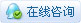12600浏览

# [入门教程] 写给设计师的趣味编程指南－（4）​让图形跑起来（下）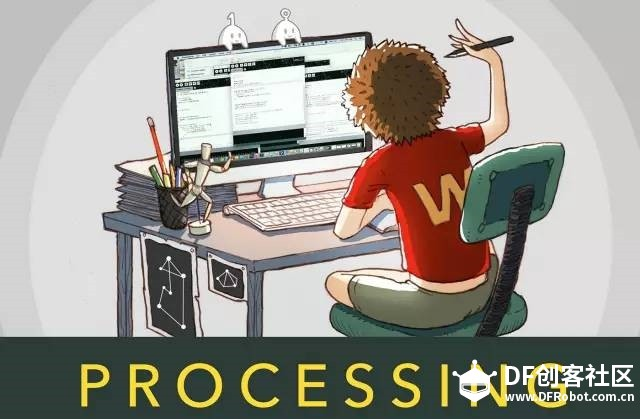运动与函数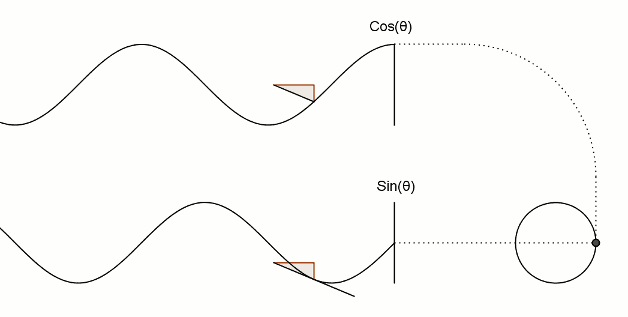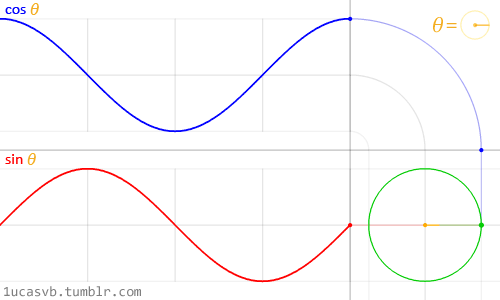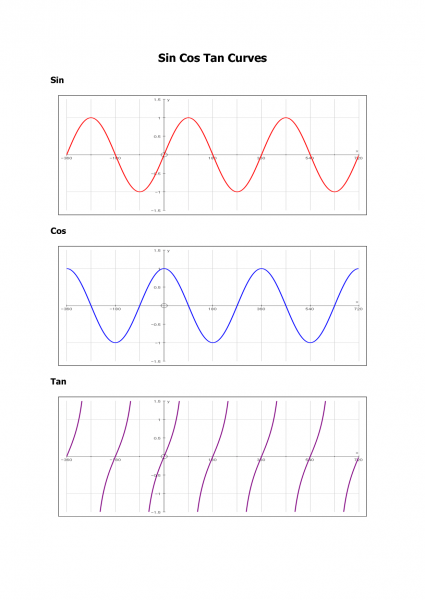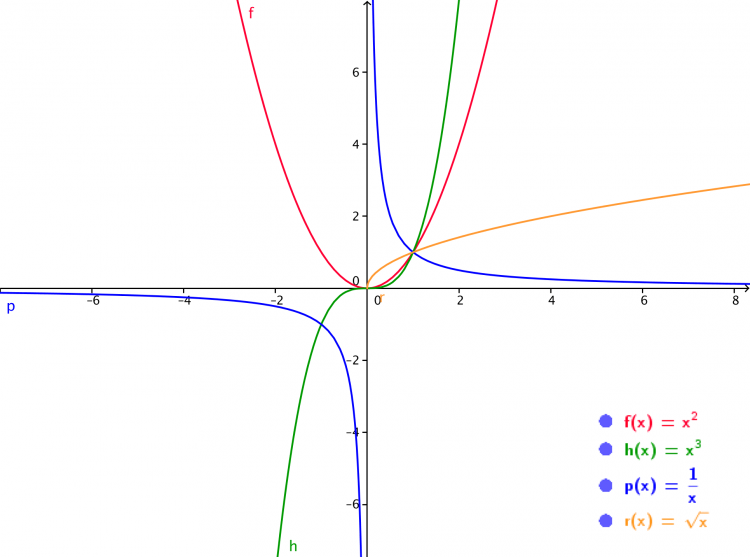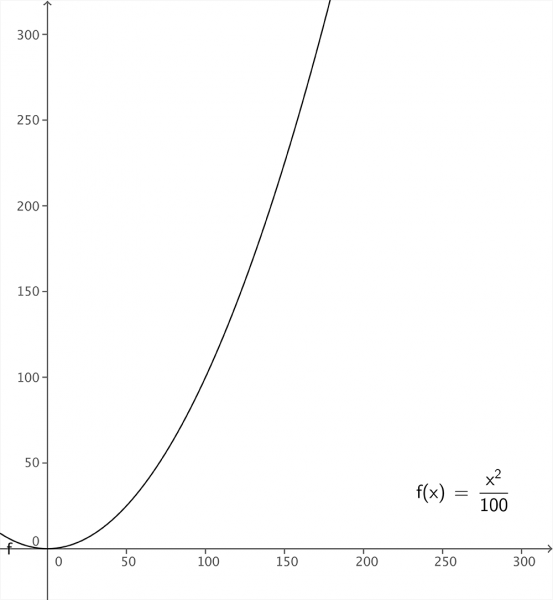[mw_shl_code=applescript,true]float x, y;
void setup(){
size(300, 300);
background(0);
x = 0;
}
void draw(){
stroke(255);
strokeWeight(2);
y = pow(x, 2) / 100.0; //pow函数会返回指定数的n次方（x,2）代表 x的平方，第一个参数是底数，第二个参数代表指数
point(x, y);
x++;
}[/mw_shl_code]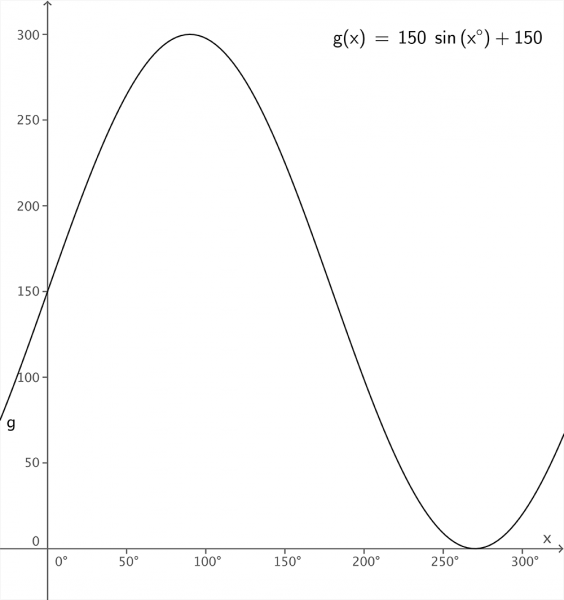[mw_shl_code=applescript,true]float x,y;
void setup(){
size(300, 300);
background(0);
x = 0;
}
void draw(){
x++;
stroke(255);
strokeWeight(2);
point(x, y);
}[/mw_shl_code][td]
 函数名 作用 格式 abs 返回指定数的绝对值 abs（x) log 返回以自然对数 e 为底，n 的对数 log(n) sq 返回指定数的平方根 sq(x) sqrt 返回指定数的平方根 sqrt(x) pow 返回指定数的 n 次方 pow(x,n) exp 返回自然对数 e 的 n 次方 exp(n)

y = x²  →   y = pow(x, 2)  或 y = sq(x)
y = x³  →   y = pow(x, 3)
y = xⁿ  →   y = pow(x, n)
y = 4ⁿ  →   y = pow(4, n)
y ＝logₑ² →  y = log(2)
y = e² → y = exp(2)
y = √5 → y = sqrt(5)

[td]
 函数名 作用 格式 sin 正弦函数 sin（x) cos 余弦函数 cos（x) tan 正切函数 tan（x) radians 将角度值转化成弧度值 raidans(x) degrees 将弧度值转化为角度值 degrees(x)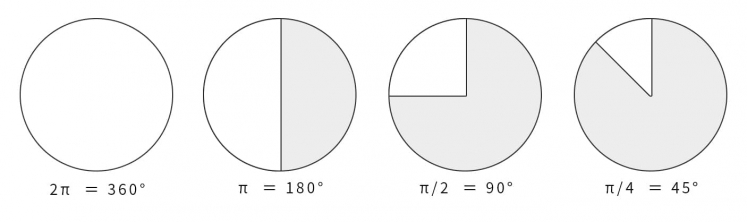degrees函数的作用恰恰相反，可以将弧度值转化为角度值。在编辑区内直接输入  print(degrees(PI/2));   看看结果会是多少？

[mw_shl_code=applescript,true]float x, y;
void setup(){
size(700, 300);
}
void draw(){
background(234, 113, 107);
y = sin(radians(x)) * 150 + 150;
x++;
noStroke();
ellipse(x, y, 50, 50);
}[/mw_shl_code]sin函数是周期函数，最小值是－1，最大值为 1 。屏幕高度为 300 。根据 y = sin(radians(x)) * 150 + 150 ，因此 y 值的变化范围就会刚好控制在 0 到 300 之内。[mw_shl_code=applescript,true]float x, y, r, R, angle;
void setup(){
size(300, 300);
r = 20; //圆的直径
R = 100; //运动轨迹的半径
x = 0;
angle = 0;
y = height/2;
}
void draw(){
background(234, 113, 107);
translate(width/2, height/2); //将原点移至屏幕中心
noStroke();
x = R *cos(angle);
y = R * sin(angle);
ellipse(x, y, r, r);
angle += 0.05;
}[/mw_shl_code]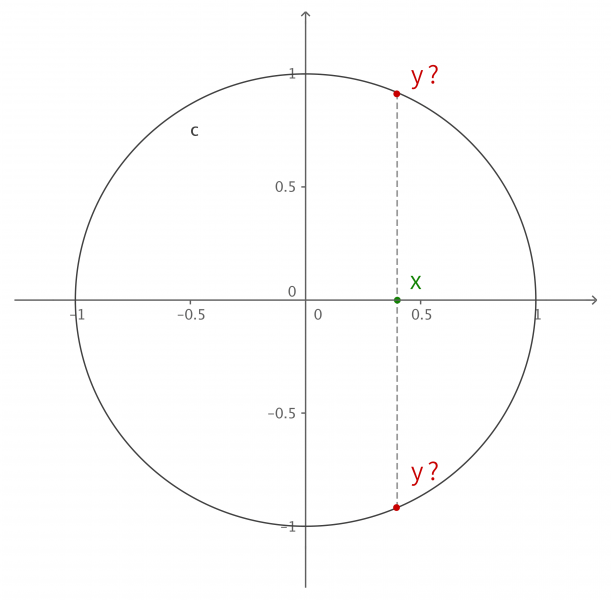y = (包含x的神秘表达式？) ；
x＋＋ ；

x = R * cos(angle);
y = R  * sin(angle);
angle += 0.05;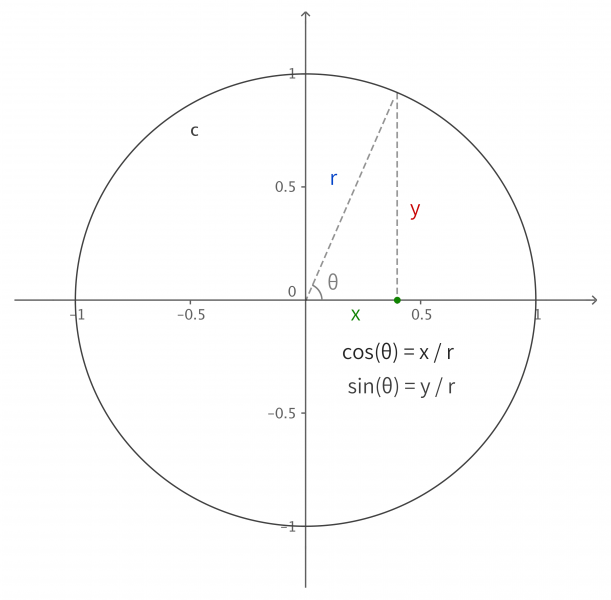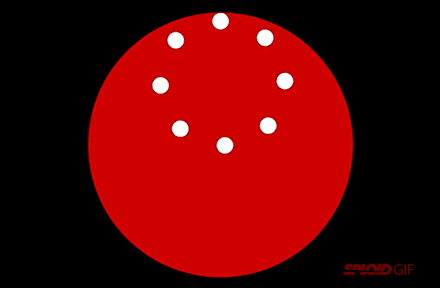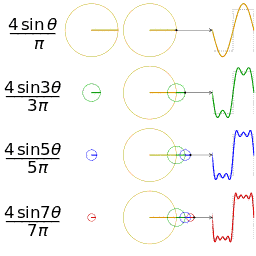[td]
 函数 作用 tranlate（x, y) 平移坐标系 scale(a) 缩放坐标系 rotate(a) 旋转坐标系 pushMatrix（）／ popMatrix（） 变换堆栈（存取坐标系）
translate函数
translate函数前面有提到过，用于平移图形的坐标系

translate(a, b)

• 对比两段代码，看有何不同（ 为简化代码，可以不输入size函数，屏幕的宽高为默认值 100 ）

ellipse(0, 0, 20, 20);translate(50, 50);ellipse(0, 0, 20, 20);rotate函数

rotate(a)

ellipse(50, 50, 20, 20);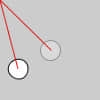scale函数

scale(a)

ellipse(0, 0, 20, 20);scale(4);ellipse(0, 0, 20, 20);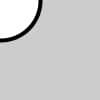scale(4，2);ellipse(0, 0, 20, 20);

void setup(){
size(300, 300);
r = 20; //圆的直径
R = 100; //运动轨迹的半径
}
void draw(){
background(234, 113, 107);
translate(width/2, height/2); //将原点移至屏幕中心
rotate(angle);
noStroke();
ellipse(0 ,R ,r ,r);
angle += 0.05;
}[/mw_shl_code]pushMatrix();    //保存坐标系状态
translate(50, 50);
ellipse(0, 0, 20, 20);
popMatrix();     //读取坐标系状态
rect(0, 0, 20, 20);

translate(40, 10);translate(10, 40);ellipse(0, 0, 20, 20);

translate(50, 50);ellipse(0, 0, 20, 20);

ellipse(50, 50, 50, 20);

translate(50, 50);rotate(radians(45));ellipse(0, 0, 50, 20);   //为了看出旋转的角度变化，绘制一个椭圆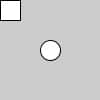pushMatrix();

pushMatrix();
…
popMatrix();
…
popMatrix();
• 为了更直观地表明对应关系，采取了缩进的形式

[mw_shl_code=applescript,true]int x, y;
float angle;
void setup(){
size(300, 300);
background(234, 113, 107);
noStroke();
x = 0; //当需要 x 的初始值为 0 的时候。可以不写这句代码。在声明变量时，默认值即是零
y = 0; //同上
angle = 0; //同上
}
void draw(){
angle += 0.25;
y—;
translate(width/2, height/2);
pushMatrix();
rotate(angle);
ellipse(x, y, 5, 5);
popMatrix();
}[/mw_shl_code][mw_shl_code=applescript,true]float x, y, angle;
void setup(){
size(300, 300);
background(234, 113, 107);
noStroke();
}
void draw(){
angle += 0.01;
x = sin(angle) *100;
y = cos(angle) * 100;
translate(width / 2, height / 2);
pushMatrix();
scale(1 + 0.1 * sin(angle * 10));
ellipse(x, y, 5, 5);
popMatrix();
}[/mw_shl_code][mw_shl_code=applescript,true]可别被它欺骗了，圆点其实只在做圆周运动。这个坐标系的缩放用摄像头去类比会较易理解，一个不断前后运动的摄像头在拍摄一个作圆周运动的点。[mw_shl_code=applescript,true]float x1, y1, x2, y2, r, R;
float angle1, angle2;
void setup(){
size(300, 300);
r = 12;
R = 120;
angle1 = 0;
angle2 = PI/4;
}
void draw(){
background(234, 113, 107);
noStroke();
translate(width / 2, height / 2);
angle1 += 0.02;
angle2 += 0.06;
x1 = R *sin(angle1);
y1 = R *cos(angle1);
x2 = R/2 *sin(angle2);
y2 = R/2 *cos(angle2);
ellipse(x1, y1, r/2, r/2);
ellipse(x2, y2, r, r);
ellipse(-x1, -y1, r/2, r/2);
ellipse(-x2, -y2, r, r);
ellipse(x1, -y1, r/2, r/2);
ellipse(x2, -y2, r, r);
ellipse(-x1, y1, r/2, r/2);
ellipse(-x2, y2, r, r);
stroke(255);
strokeWeight(3);
line(x1, y1, x2, y2);
line(-x1, -y1, -x2, -y2);
line(x1, -y1, x2, -y2);
line(-x1, y1, -x2, y2);
}[/mw_shl_code]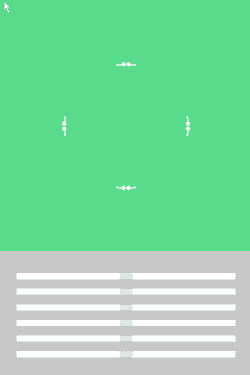END

[mw_shl_code=applescript,true]float browX, earD, eyeD, faceD;
void setup(){
size(500, 500);
}
void draw(){
background(200, 0, 0);
browX = 150 + sin(frameCount / 30.0)* 20;
earD = 180 + sin(frameCount / 10.0) *20;
eyeD = 60 + sin(frameCount/30.0) *50;
faceD = 300;
strokeWeight(8);
ellipse(175, 220, earD, earD);
ellipse(width - 175, 220, earD, earD);
rect(100, 100, faceD, faceD);
line(browX, 160, 220, 240);
line(width-browX, 160, width-220, 240);
fill(random(255),random(255),random(255));
ellipse(175, 220, eyeD, eyeD);
ellipse(width-175, 220, eyeD, eyeD);
fill(255);
point(width/2, height/2);
triangle(170 - cos(frameCount / 10.0) * 20, 300 - sin(frameCount / 10.0) * 20, width - (170 + cos(frameCount / 10.0) * 20), 300 + sin(frameCount / 10.0) * 20, 250, 350);
}[/mw_shl_code]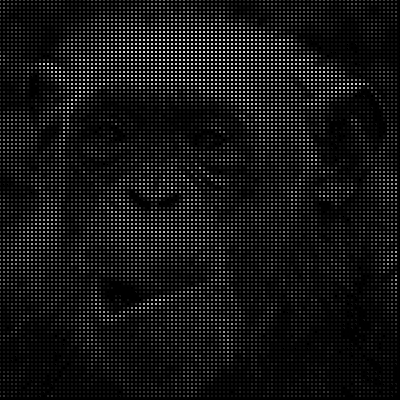### luna初级技神

 继续拜读中~~~

 这个帖子太好了，数学老师炒鸡喜欢### rongrjianxin初级技师

 虽然我是计算机专业的，但是看完后我想把从小学到大学的数学再学一遍### Sipang高级技师

 厉害了，我的哥### svw初级技匠

 2 个问题 1. 图中gif都是process画的？ 2. 啥事介绍下bezier曲线和b-spline，T-spline。那个更好玩。### kaka高级技师 楼主|

 svw 发表于 2017-1-27 22:19 2 个问题 1. 图中gif都是process画的？ 2. 啥事介绍下bezier曲线和b-spline，T-spline。那个更好玩。 ... 1. 图中gif都是process画的？ processing可以生成图片，然后用PS软件做成gif,还有一种方法用插件，回头我会做个相关教程### kaka高级技师 楼主|

 svw 发表于 2017-1-27 22:19 2 个问题 1. 图中gif都是process画的？ 2. 啥事介绍下bezier曲线和b-spline，T-spline。那个更好玩。 ... 2. 啥事介绍下bezier曲线和b-spline，T-spline。那个更好玩。 关于其他曲线，方法都告诉你了，其实也大同小异而已### gray6666初级技神

 请问程序开源吗 ？在哪里可以下载？### kaka高级技师 楼主|

 gray6666 发表于 2017-2-22 16:08 请问程序开源吗 ？在哪里可以下载？ 你是问processing程序吗？给你一个下载地址http://www.makerspaces.cn/forum. ... p;tid=14&extra=### www.sxzisha.com学徒

 好帅气啊### DFrJ0Q538pQ学徒

 感谢科普！！processing真的是太神奇了

 最后一张图的效果改怎么做呢

 您需要登录后才可以回帖 登录 | 立即注册 本版积分规则 回帖并转播 回帖后跳转到最后一页

[[wsData.name]]

#### 硬件清单

• [[d.name]]#### 楼主的其它帖子